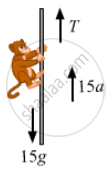# A Monkey of Mass 15 Kg is Climbing a Rope Fixed to a Ceiling. If It Wishes to Go up with an Acceleration of 1 M/S2, How Much Force Should It Apply on the Rope? If the Rope is 5 M Long - Physics

Sum

A monkey of mass 15 kg is climbing a rope fixed to a ceiling. If it wishes to go up with an acceleration of 1 m/s2, how much force should it apply on the rope? If the rope is 5 m long and the monkey starts from rest, how much time will it take to reach the ceiling?

#### Solution

Mass of the monkey, m = 15 kg,
Acceleration of the monkey in the upward direction, a = 1 m/s2
The free-body diagram of the monkey is shown below:From the free-body diagram,
T − [15g + 15(a)] = 0
T − [15g + 15(1)] = 0
⇒ T = 5 (10 + 1)
⇒ T = 15 × 11 = 165 N
The monkey should apply a force of 165 N to the rope.

Initial velocity, u = 0
s = 5 m
Using, $s = ut + \frac{1}{2}a t^2$, we get:

$5 = 0 + \left( \frac{1}{2} \right) \times 1 \times t^2$

$\Rightarrow t^2 = 5 \times 2$

$\Rightarrow t = \sqrt{10} s$
Hence, the time required to reach the ceiling is $\sqrt{10} s$

Concept: Newton’s Second Law of Motion
Is there an error in this question or solution?

#### APPEARS IN

HC Verma Class 11, Class 12 Concepts of Physics Vol. 1
Chapter 5 Newton's Laws of Motion
Q 36 | Page 82